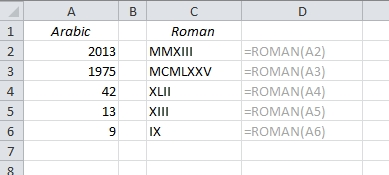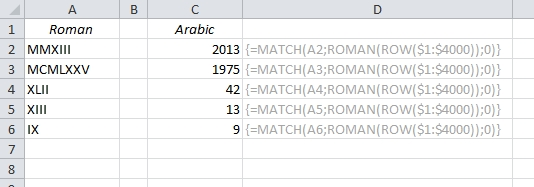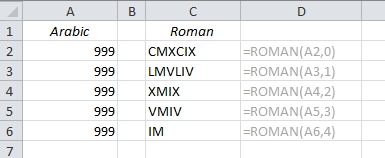# Use Excel to convert Arabic Numbers to Roman and back

Luckily, we don’t use the roman numerals much today, so this post is just for fun! Converting an Arabic number (normal number) into a Roman numeral is one of the easiest things you can do in Excel, but converting back from Roman to Arabic is equally difficult. Let’s have a look:

## Convert to Roman numerals

If you want to convert a normal number into a classic Roman numeral, there is a built-in function in Excel: ROMAN## Convert a Roman number into an Arabic number

But what about the other way around? What if you see one of these cryptic Roman numbers and you want to convert it back into a normal English, or rather, Arabic, number? There is no Excel function for that, so we have to find a work-around:

{=MATCH(A2,ROMAN(ROW(\$1:\$5000)),0)}As you can tell by the curly brackets, this is an array formula. The brackets appear in the formula field when you close the formula with Ctrl+Shift+Enter. Here’s how it works:

• ROW(\$1:\$4000) generates a list of row numbers, from 1 to 4000*: {1,2,3,4,5,6,…}
• When you wrap ROMAN around it, the list looks like this: {“I”,”II”,”III”,”IV”,”V”,”VI”,…}
• The whole formula starts with MATCH(A2,, which is a command to look up the value in A2 (in this case “MMXIII”) in the array and return the position of it. As we saw above, the lookup array is {“I”,”II”,”III”,”IV”,…}, and “MMXIII” will be the 2013th value in this sequence.
• The zero in the end of the formula tells Excel to find an exact match.

Note: Apparently, the Romans couldn’t agree on one uniform number system, so you can actually see several different versions of it. The Excel function allows you to use a second argument to calculate the different types. I am unsure if anyone has ever used it, though…* Why 4000? The ROMAN function only works with numbers from 1 to 3999!

More Excel oddities:

Are you using a non-English version of Excel? Click here for translations of the 100 most common functions.